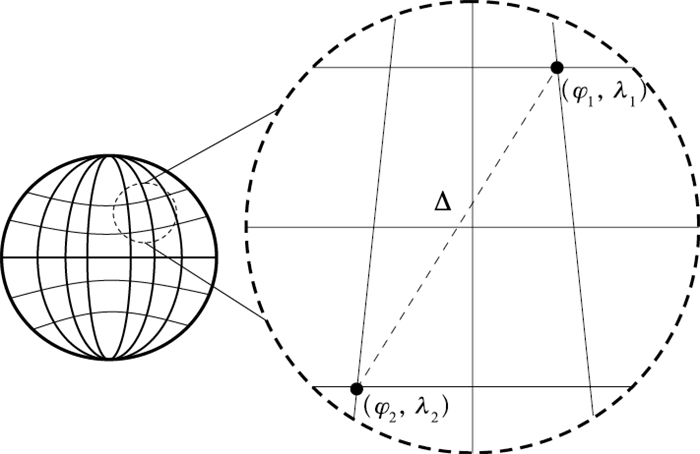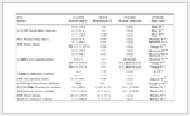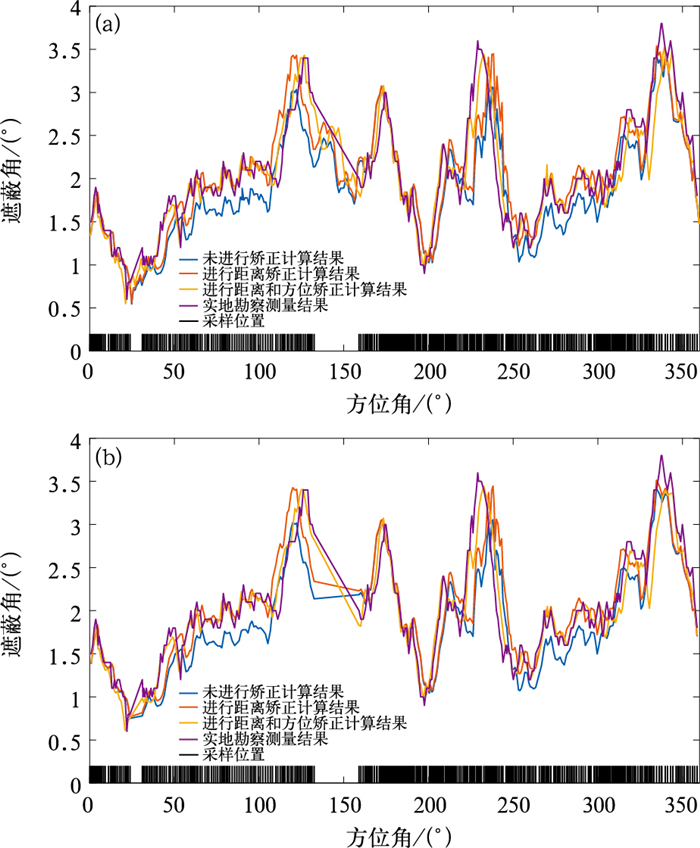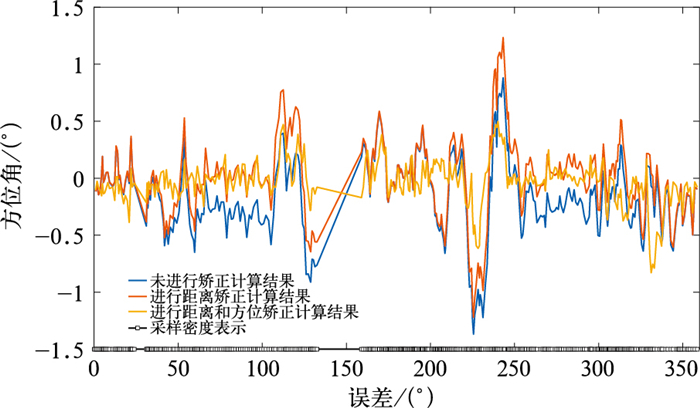﻿ 基于SRTM天气雷达净空环境评估算法误差分析
 快速检索 中文标题 英文标题 作者中文名 作者英文名 单位中文名 单位英文名 中文关键词 英文关键词 中文摘要 英文摘要 基金项目 全文
 气象2020, Vol. 46Issue (2): 278-282.  DOI: 10.7519/j.issn.1000-0526.2020.02.013### 引用本文 [复制中英文]

[复制中文]
JING Haoran, ZHENG Wei, LIU Zongqing, et al, 2020. Error Analysis on the Assessment Algorithm of Clearance Environment of Weather Radar Based on SRTM[J]. Meteorological Monthly, 46(2): 278-282. DOI: 10.7519/j.issn.1000-0526.2020.02.013.
[复制英文]

### 文章历史

2018年10月15日收稿
2019年9月16日收修定稿

1. 四川省气象探测数据中心, 成都 610072
2. 中国气象局大气探测重点开放实验室, 成都 610225
3. 高原与盆地暴雨旱涝灾害四川省重点实验室, 成都 610072

Error Analysis on the Assessment Algorithm of Clearance Environment of Weather Radar Based on SRTM
JING Haoran1,2,3, ZHENG Wei2,3, LIU Zongqing2,3, XIE Xiaolin2,3, PENG Tao2,3
1. Sichuan Meteorological Observation and Data Center, Chengdu 610072;
2. CMA Key Laboratory of Atmospheric Sounding, Chengdu 610225;
3. Heavy Rain and Drought-Flood Disasters in Plateau and Basin Key Laboratory of Sichuan Province, Chengdu 610072
Abstract: Shuttle Radar Topography Mission (SRTM) data were used to evaluate the clearance environment and the site-selection evaluation efficiency of radar was effectively improved. In this paper,the comparative experiment of weather radar clearance environment assessment is carried out by using manual measurement data and SRTM data. Error analysis of this method suggested that the evaluation algorithm of weather radar clearance environment based on SRTM was matching with the artificial measured data. The calculation error was significantly reduced when corrected with azimuth correction and distance correction algorithms.
Key words: SRTM (Shuttle Radar Topography Mission)    weather radar    clearance environment    error analysis

1 算法与数据图 1 经纬度计算方位及距离示意图 Fig. 1 Computed azimuth and distance of longitude and latitude

 \begin{aligned} \Delta=& 111.199 \\ & \sqrt{\left(\varphi_{1}-\varphi_{2}\right)^{2}+\left(\lambda_{1}-\lambda_{2}\right)^{2} \cos ^{2}\left(\frac{\varphi_{1}+\varphi_{2}}{2}\right)} \end{aligned} (1)

 $\frac{投影上纬线长度}{地球上实际纬线长度}=\frac{2 \pi R}{2 \pi R \cos \varphi}=\sec \varphi$ (2)
 $\mathrm{d} y=R \cdot \mathrm{d} \lambda$ (3)
 $\mathrm{d} x=R \cdot \sec \varphi \cdot \mathrm{d} \varphi$ (4)
 $\theta=\tan ^{-1}(\mathrm{d} x / \mathrm{d} y)$ (5)

2 对比分析表 1 雷达净空环境评估方法对比 Table 1 Comparison of radar clearance environment assessment methods表 2 站点A基本信息 Table 2 Basic information on Site A图 2 未抽样进行距离和方位校正算法(a)，抽样后进行距离和方位校正算法(b)计算结果与实测结果对比 Fig. 2 Comparison of the results calculated and the measured results (a) unsampled distance and azimuth correction algorithm, (b) distance and azimuth correction algorithm after sampling图 3 站点A计算值与实测值误差 Fig. 3 Error diagram of calculated value and measured value for Site A表 3 计算值误差分析 Table 3 Calculation parameters and error analysis
3 结论与讨论